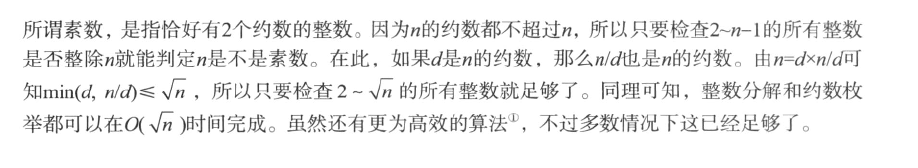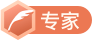# Miller__Rabin素数测试

如果  n 是素数 且与a 互质 那么则有  a ^(n -1)  = 1 (mod n)

1、因此首先可以进行快速幂取模函数  qpow()
2 、先将y(y = n-1) 的偶数倍先将其右边为0 的部分去掉 ,然后进行快速幂求模
3、 这里求出的结果可能不会是1  因此将n 适当的放大  x= (x*x)%n  y 也同时扩大1倍 这里的目的其实就是为了在求快速幂当中取模 过程中模出现为1 或者n-1的情况
4、 最后的结果如过 x = n - 1或者 y 是奇数的话，说明已经通过了测试#include<iostream>
using namespace std;

int qpow(int a, int b , int M)
{
//快速幂取模
int ans = 1;
while(b)
{
if(b&1)
{
ans*= a;
ans %= M;
}
a*= a ;
a%= M;
b>>= 1;
}
return ans;
}
bool Miller_Rabin(int x , int n)
{
//这里主要是测试n 是不是素数
int y = n -1;
while(!(y&1)) y>>= 1 ;//去掉y 右边全是0的部分
x = qpow(x, y, n);  //快速幂取模x 为底 y 为幂 n 为模
while(y < n-1 && x != 1&& x != n-1)
{
x = (x*x)%n ;
y <<= 1;
}
//最后的结果是要么x = n -1 或者是 为奇数

return x == n-1 || (y&1) == 1;
}
int isPrime32(int n )
{
//在32 位下， 如果能以2 7 61 为底通过Miller_Rabin测试那么就是素数了
if(n == 2 || n == 7 || n == 61) return 1;
if(n == 1 || (n&1) == 0) return 0 ; //如果n为1 或者说是偶数 那么一定不是合数
return  Miller_Rabin(2, n) && Miller_Rabin(7,n) && Miller_Rabin(61, n);
}
int main()
{

int n ;
while(cin >>n)
{
if(isPrime32(n))
{
cout<<"是素数"<<endl;
}
else
{
cout<<"不是素数"<<endl;
}
}
return 0 ;
}


wangxiaomingCSDN认证博客专家 架构 Spring Boot Redis

09-021万+
11-05855009-022880
06-19124
12-05413
05-241万+
09-04585
08-07537
07-096257
03-10560
©️2020 CSDN 皮肤主题: Age of Ai 设计师:meimeiellie点击重新获取扫码支付1.余额是钱包充值的虚拟货币，按照1:1的比例进行支付金额的抵扣。
2.余额无法直接购买下载，可以购买VIP、C币套餐、付费专栏及课程。余额充值